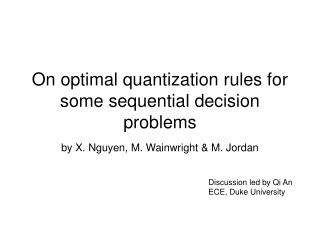# On optimal quantization rules for some sequential decision problems - PowerPoint PPT PresentationDownload PresentationOn optimal quantization rules for some sequential decision problems

Download Presentation## On optimal quantization rules for some sequential decision problems

- - - - - - - - - - - - - - - - - - - - - - - - - - - E N D - - - - - - - - - - - - - - - - - - - - - - - - - - -
##### Presentation Transcript

1. On optimal quantization rules for some sequential decision problems by X. Nguyen, M. Wainwright & M. Jordan Discussion led by Qi An ECE, Duke University

2. Outline • Introduction • Background • Approximate design • Suboptimality of stationary designs • Discussion

3. Introduction • What’s decentralized detection problem? • A hypothesis problem in which the decision maker have no direct access to the raw data, but instead must infer based only on a set of local quantization functions and a sequence of summary statistics. • What’s the goal? • The goal is to design both the local quantization functions and to specify a global decision rules so as to predict the underlying hypothesis H in a manner that optimally trades off accuracy and delay

4. Introduction • For general framework of sequential decentralized problems, Veeravalli defined five problems (case A to E) distinguished by amount of information available to the local sensors. • Veeravalli use likelihood ratio test to solve the case E, where each local sensor has its current data, On, as well as the summary statistics form all of the other local sensor.

5. In Veeravalli’s paper, he conjectured that stationary local decision functions may actually be optimal for case A, where neither local memory nor feedback are assumed to be available. In this paper, the author shows that stationary decision functions are, in fact, not optimal for decentralized problem of case A. O1n …… Otn Usually, O∈{1,…,M} and U∈{1,…,K}, where M>>K S1 St U1n=φn(O1n) Utn=φn(Otn) quantization functions S0 fusion center Ĥ=γn(U11,…,Ut1,U12,…,Ut2,…,U1n,…,Utn) decision rule Let Xn=[O1n,…,Otn] and Zn=[U1n,…,Utn] .

6. Sequential detection • Consider a general centralized sequential detection problem. Let P0 and P1 represent the class-conditional distributions of Z, when conditioned on {H=0} and {H=1}, and and are corresponding density functions. • Focusing the Bayesian formulation, we let and denote the prior probabilities of the two hypothesis • The cost function we want to minimize is a weighted sum stopping time cost per step We want to choose the pair so as to minimized the expected loss

7. Dynamic programming • The previous centralized sequential detection can be solved by DP approach by iteratively updating the cost function over horizon. • But it is not straightforward to apply DP approach to decentralized versions of sequential detection.

8. Wald’s approximation The optimal stopping rule for the cost function takes the form: The optimal decision rule has the form: Let’s define two types of errors

9. Wald’s approximation The cost function of the decision rule based on envelop a and b can be written as where and It is hard to calculate this cost function but it can be easily approximated. The errors α,β are related to a and b by the classical inequalities

10. Wald’s approximation If we ignore the overshoot and replace the inequalities with the equalities, i.e. we can therefore get the approximate cost function: where

11. Approximate design • Now consider the decentralized setting. Given a fixed stationary quantizer φ, Wald’s approximation suggests the following strategy: • For a given set of admissible errors α and β, first assign the values of thresholds and • Then use the quantity G(α,β) as an approximation to the true cost J(a,b). By assuming , the author proves

12. Suboptimallity of the stationary design • It was shown by Tsitsiklis that optimal quantizers φn take the form of threshold rules based on the likelihood ratio • Veeravalli asked whether these rules can be taken to be stationary, a problem that has remained open.

13. Suboptimallity of the stationary design • A simple counterexample to show the optimal quantizer is not stationary Consider a problem in which and the conditional distributions are Suppose the prior are and , and the cost for each step is If we restrict to binary quantizers, then there are only three possible quantizers 1. Design A: . As a result, the pdf for Zn is 2. Design B: . As a result, the pdf is 3. Design C: . As a result, the pdf is

14. Suboptimallity of the stationary design Where * is corresponding to the nonstationary design by applying design A for only the first step and applying the design B for the remaining steps.

15. Asymptotic suboptimality of stationary designs As we can see in the approximate cost function, it is composed of two KL divergences. If we want to achieve a small cost we need to choose a quantizer φ which make both divergences, μ1 and μ0, as large as possible.

16. Discussion • The problem of decentralized sequential detection encompasses a wide range of problems involving different assumptions about the memory and feedback. • They have provided an asymptotic characterization of the cost of the optimal sequential test in the setting of case A. • They provided an explicit counterexample to the stationary conjecture and showed that under some conditions there is a guaranteed range of prior probabilities for which stationary strategies are suboptimal.